Share this: Google+

## Simultaneous Rotation using Excel

Using matrix notation we get advantage that we do not need to type the formula for each point one by one. All we need to do is to use matrix multiplication. This simplifies the job if we have many points.

As numerical example, we have ten points, each with their coordinate in 2 dimension and you want to rotate those 10 points simultaneously from the mean of these coordinate. Suppose we set the rotation angle is 90 degrees we wish get the result as the following figure.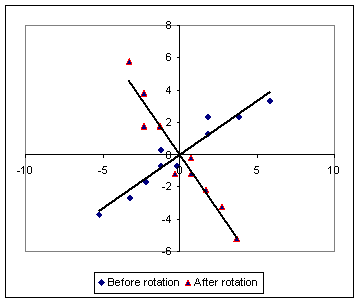Suppose the matrix coordinate data is calledThe first column is the x- coordinate and the second column is the y- coordinate. Because we want to rotate those points about the center of all points, we need to calculate the mean coordinate.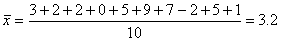,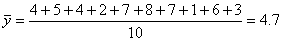Thus, we subtract the first column of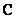with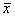and subtract the second column ofwith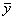to translate the coordinate to the center of all points. The results isOur rotation matrix is given by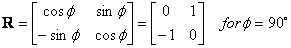Multiply this rotation matrix with the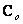, we get the new coordinate of rotated points simultaneously.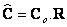The problem now is how to do that in Microsoft Excel using matrix function? You can simply use MMULT excel function. Note that if you define your own matrix or array, you need to select in it a range (by mouse drag), then press F2 then Ctrl-Shift-Enter.

Preferable reference for this tutorial is

Teknomo, Kardi (2015) Rotation of Points Simultaneously Using Microsoft Excel Matrix. https:\\people.revoledu.com\kardi\ tutorial\Excel\SimultaneousRotation.html